Ex 5.3

Chapter 5 Class 8 Squares and Square Roots
Serial order wise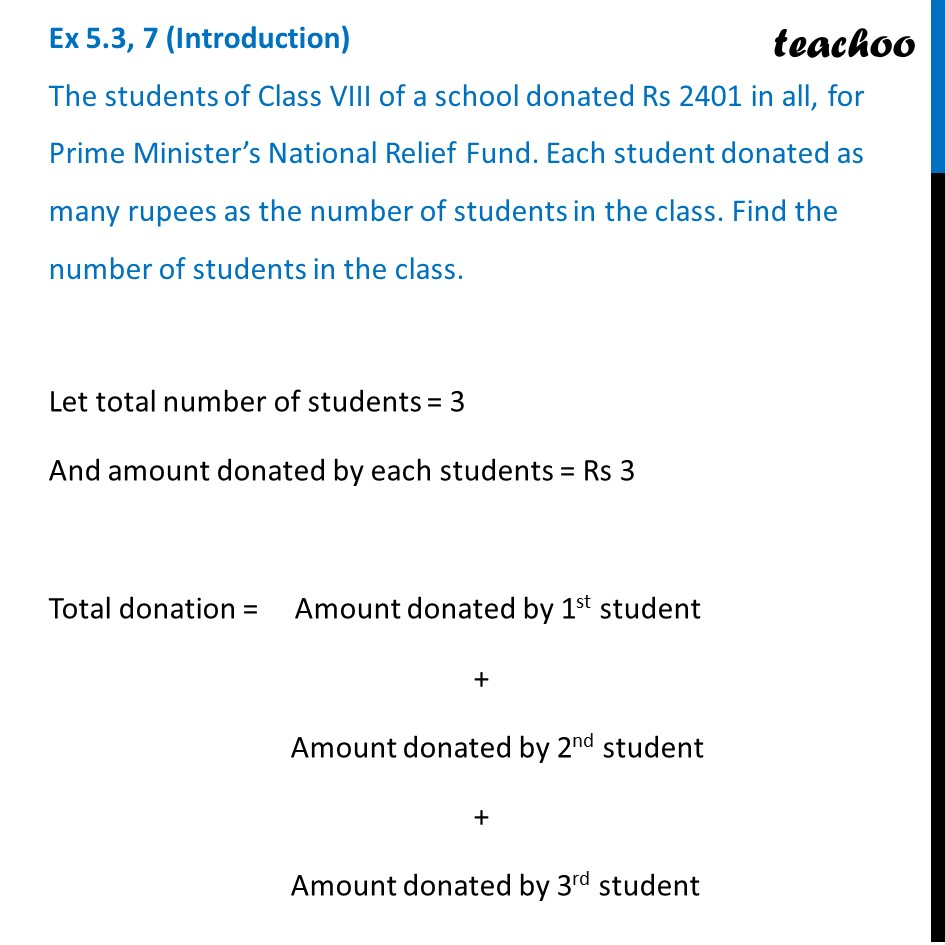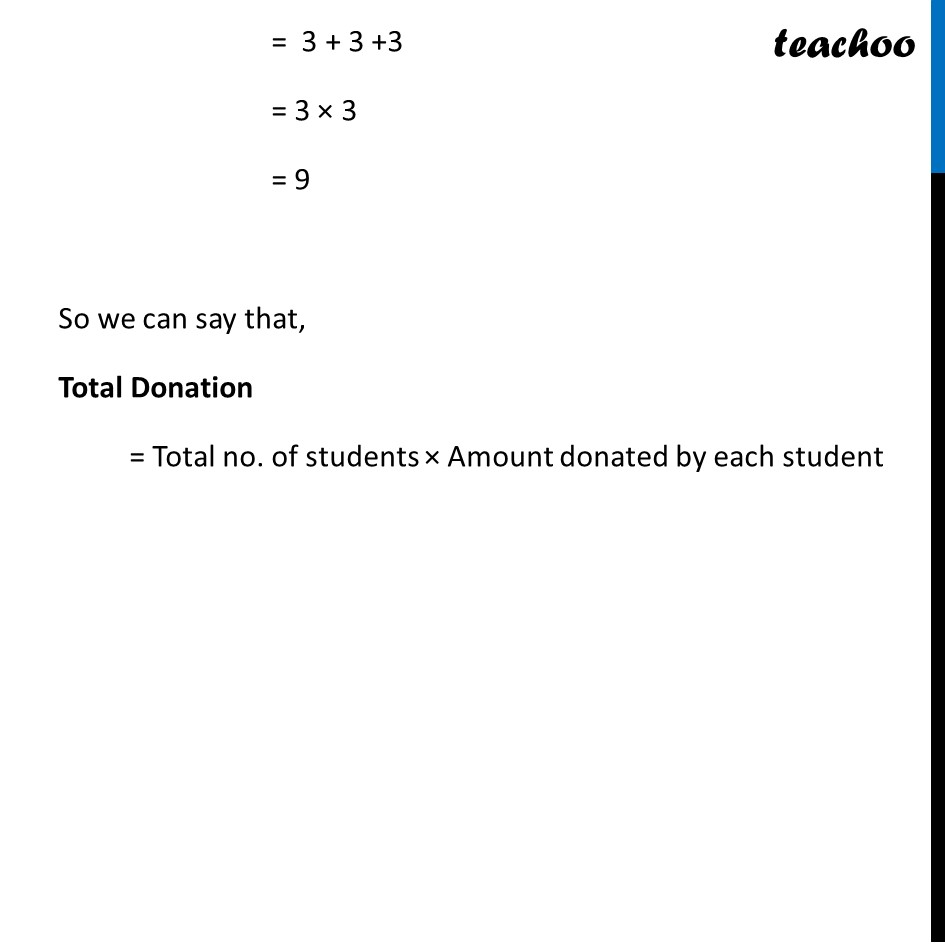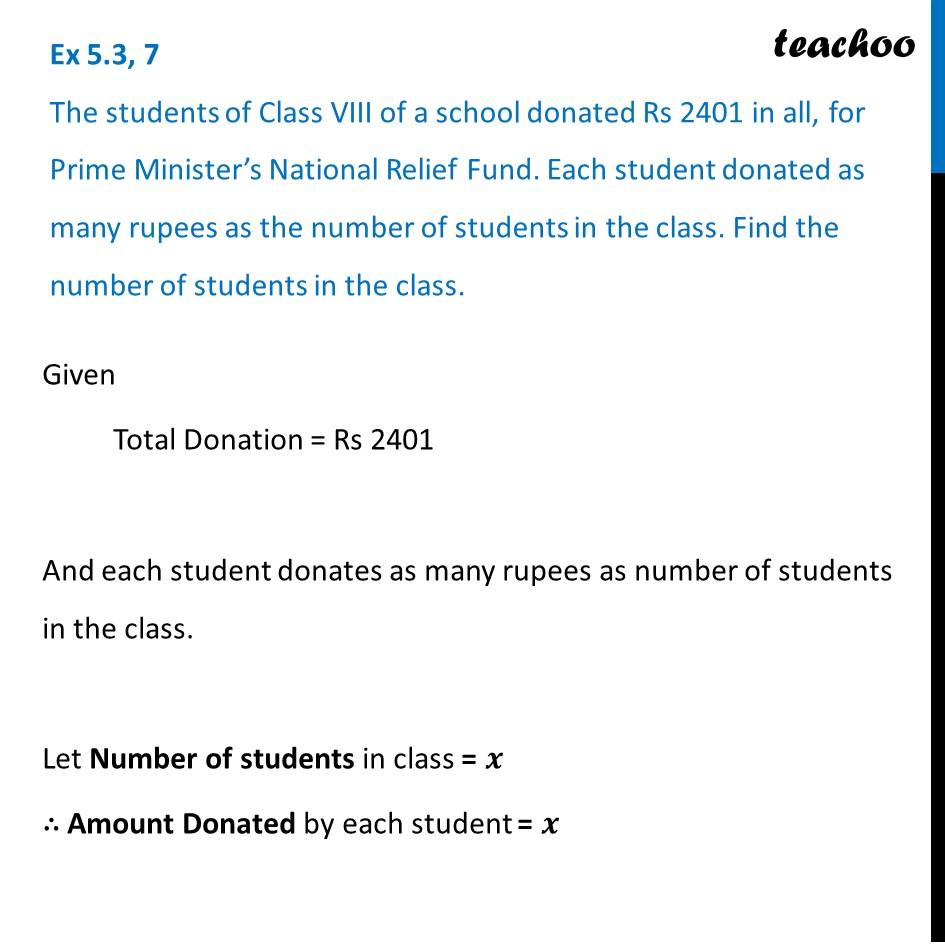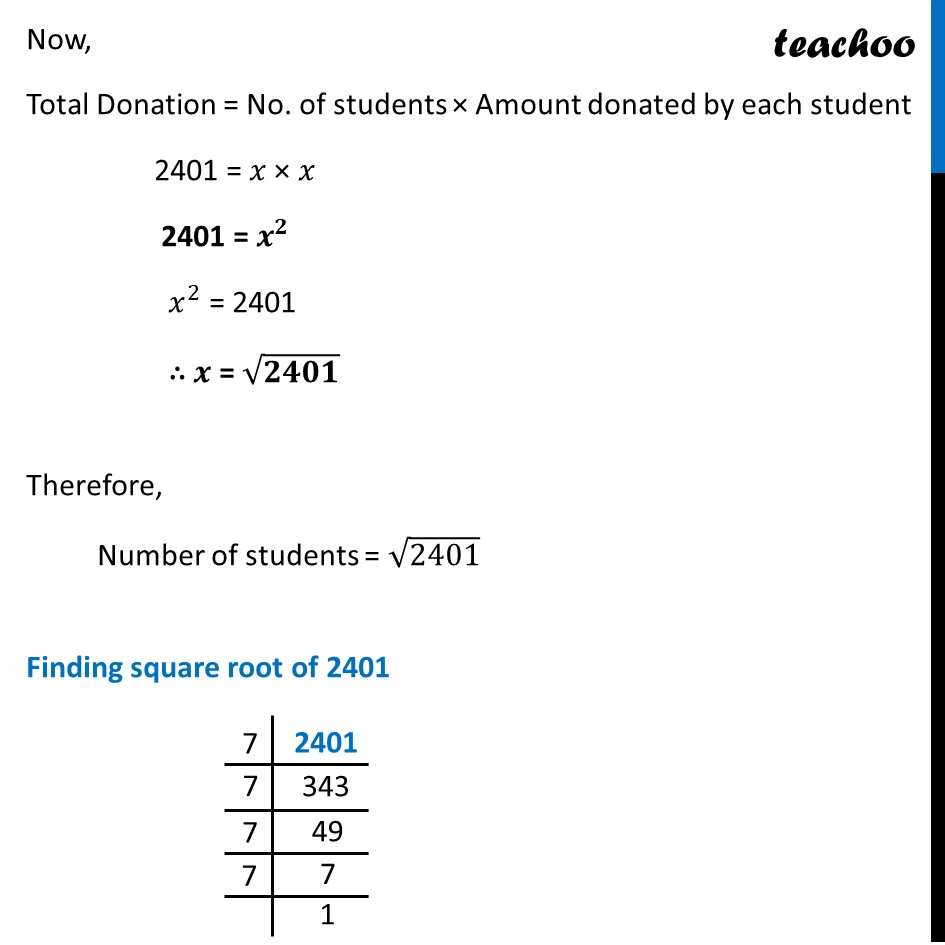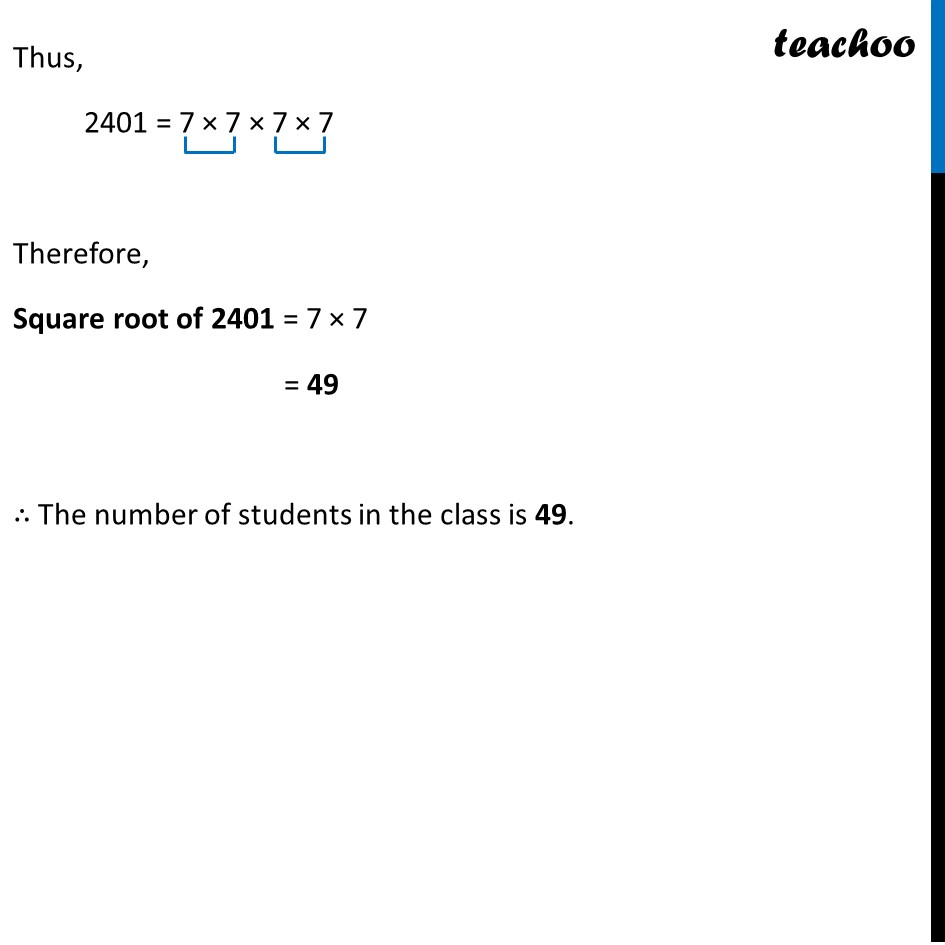Learn in your speed, with individual attention - Teachoo Maths 1-on-1 Class

### Transcript

Ex 5.3, 7 (Introduction) The students of Class VIII of a school donated Rs 2401 in all, for Prime Minister’s National Relief Fund. Each student donated as many rupees as the number of students in the class. Find the number of students in the class.Let total number of students = 3 And amount donated by each students = Rs 3 Total donation = Amount donated by 1st student + Amount donated by 2nd student + Amount donated by 3rd student = 3 + 3 +3 = 3 × 3 = 9 So we can say that, Total Donation = Total no. of students × Amount donated by each student Ex 5.3, 7 The students of Class VIII of a school donated Rs 2401 in all, for Prime Minister’s National Relief Fund. Each student donated as many rupees as the number of students in the class. Find the number of students in the class. Given Total Donation = Rs 2401 And each student donates as many rupees as number of students in the class. Let Number of students in class = 𝒙 ∴ Amount Donated by each student = 𝒙 Now, Total Donation = No. of students × Amount donated by each student 2401 = 𝑥 × 𝑥 2401 = 𝒙^𝟐 𝑥^2 = 2401 ∴ 𝒙 = √𝟐𝟒𝟎𝟏 Therefore, Number of students = √2401 Finding square root of 2401 Thus, 2401 = 7 × 7 × 7 × 7 Therefore, Square root of 2401 = 7 × 7 = 49 ∴ The number of students in the class is 49.